## MATHEMATICS PAPER 1 - KCSE 2019 ALLIANCE MOCK EXAMINATION

SECTION I (50 MARKS)

Answer all the questions from this section

1. Without using a calculator evaluate (3 marks)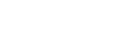1.
1. use mathematical tables to find the:
1. The square of 86.46 (1 mark)
2. The reciprocal of 27.56 (1 mark)
2. Hence or otherwise calculate the value of;  (2 marks)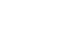1. The sum of the interior angles off an n – sided polygon is 14400. Find the value of n and hence deduce the name of the polygon. (3 marks)
1. Two containers have base areas of 750cm2 and 120cm2 Calculate the volume of the larger container in litres given that the volume of the smaller container is 400cm3. (3 marks)
1. Given that the column vectors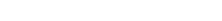and that P=2a-4b+3c . Express P as a column vector. (3 marks)
1. Solve the following inequalities and represent the range of values of x on a single number line.      (3 marks)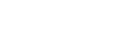1. The cost of a car outside Kenya is US \$ 4800. You intend to buy one such car through an agent who deals with Japanese Yen. The agent will charge 15% commission on the price of the car and further 72 220 Japanese Yens for shipment of the car. How many Kenya shillings will you need to send to the agent to obtain the car given that:
1 US \$ = 117.20 Japanese Yens
1US \$ = Kshs 72.34                                                                                                 (3 marks)
1. Two numbers p amd q are such that p3× q = 189 . Find p and q (3 marks)
1. Evaluate without using mathematical tables. (3 marks)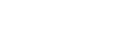1. Simplify the following expression by reducing it to a single fraction. (3 marks)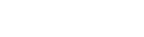1. Thirty men working at a rate of 10 hours a day can complete a job in 14 days. Find how long it would take 40 men working at the rate of 7 hours a day to complete the same job.
(3 marks)
1. The figure below shows a circle centre O and radius 6cm. sector OAB subtends an angle of 1000 at the centre of the circle as shown.Calculate to 2 decimal places the area of the shaded region. (Take π = 22/7)     (3 marks)
1. Use the prime factors of 1764 and 2744 to evaluate (3 marks)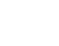1. A rectangular block is 50cm long and 15 cm wide. If its mass is 18kg and its density is 2.4g/cm3, find its height. (3 marks)
1. A triangle ABC is such that AB = 12cm, and AC = 17cm. if its area is 512cm2, find the size of angle BAC (3 marks)
1.
1. Find the greatest common divisor of the terms 9x3yand 4xy4       (1 mark)
2. Hence factorize completely the expression
9x3y- 4xy4                                                   (2 marks)

SECTION II (50 MARKS)

Answer FIVE questions ONLY from this section

1. A straight line y= 2/3x - 2/3 meets the x – axis at point T.
1. Determine the coordinates of T.                                                                        (2 marks)
2. A second line L2 is perpendicular to line L1 at T.
3. Find the equation of line L2 in the form ax+by = c where a, b and c are constants. (3 marks)
4. A third line L3 passes through (-4,1 ) and is parallel to L1. Find;The equation of line L3 in the form y=mx+c                        (2 marks)
5. The coordinates of point S at which L3 intersects L2. (3 marks)
1. A particle moves in a straight line so that its velocity is given by V=  1/2 t2-3t+7 where t is time in seconds. Find:
1. The velocity after 8 seconds.                                                                     (2 marks)
2. The acceleration when t = 0                                                                     (2 marks)
3. The minimum velocity attained. (2 marks)
4. The distance travelled in the first 2 seconds. (4 marks)
1. The points AI BI CI are the images of A(4,1), B(0,2), C(-2,4) respectively under a transformation represented by the matrix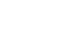.
1. Write down the coordinates of  (3 marks)
2. AII BII CII are the images of AI BI CI under another transformation whose matrix is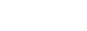. Write down the co – ordinates of AII BII CII  (3 marks)
3. Transformation M followed by N can be replaced by a single transformation P. determine the matrix for P. (2 marks)
4. Hence determine the inverse of matrix P. (2 marks)
1. The distance between two towns A and B is 460 km. a minibus left town A at 8.45 am and travelled towards Bat an average speed of 65km/hr. A matatu left B at 10.55 am on the same day and travelled towards A at an average speed of 80km/hr.
1. How far from town B did they meet? (4 marks)
2. At what time did the two vehicles meet? (2 marks)
3. A motorist started from his home at 9.15am on the same day and travelled to B at an average speed of 120km/hr. he arrived at the same time as the minibus. Calculate the distance from B to his home. (4 marks)
1. A paper cup is made in the shape of a frustum of a cone with an open top of diameter 10.5cm and a sealed bottom of diameter 7cm. it has a depth of 12cm, calculate:
1. The total surface area of the cup. (6 marks)
2. The capacity of the cup to the nearest deciliter. (4 marks)
1. The table below shows the marks scored by form four students in a mathematics test in Bidii secondary school.1. State the modal class. (1 mark)
2. Using an assumed mean of 57, calculate:
1. The mean                                                                                     (3 marks)
2. The standard deviation. (3 marks)
3. Find the mark scored by the 50th (3 marks
1. The figure below shows triangle XYZ in which line XY = 5cm, line YZ = 13.4cm and the size of angle XYZ = 57.701. Calculate the length of line XZ (4 marks)
2. Calculate the size of angle XZY (4 marks)
3. Calculate the size of angle YXZ to 4 significant figures (2 marks)
1. Four towns P,Q,R and S are such that town P is 200 km West of Q. Town R is at a distance of 80km on a bearing of 0490 from P. Town S is due East of R and due North Of Q.
1. Using a scale of 1cm to represent 20km, make an accurate scale drawing to show the relative positions of the towns. (4 marks)
2. Find:
1. Determine the bearing of S from P       (1mark)
2. Determine the distance of Q from S        (2 marks)
3. Determine the bearing of Q from R (1 mark)
4. Determine the distance of R from S      (2 marks)## MARKING SCHEME• ✔ To read offline at any time.
• ✔ To Print at your convenience
• ✔ Share Easily with Friends / Students